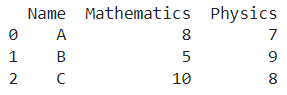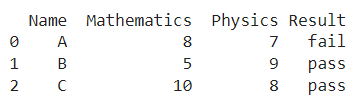# Pandas Conditional Creation Of A Series-dataframe Column

A DataFrame is the primary data structure of the Pandas library in Python and is commonly used for storing and working with tabular data.

A common operation that could be performed on such data is to conditionally create a series or dataframe column in order to add more information to the data.

To start working with Pandas, we first need to import it :

### Python 3 Code :

``import pandas as pd``

We’ll also need another library for this operation, i.e. the NumPy library. To start working with Numpy, we first need to import it :

### Python 3 Code :

``import numpy as np``

## Running Example

Let us understand this operation with the help of an example. Consider the following DataFrame containing 3 students with names A, B, and C and their corresponding marks (out of 10) for two subjects, Mathematics and Physics.Code snippet for generating the above DataFrame :

## Conditional Creation Of A Series/dataframe Column

``````import pandas as pd

# Dictionary for our data
data = {'Name' : ['A', 'B', 'C'], 'Mathematics' : [8, 5, 10], 'Physics' : [7, 9, 8]}

# DataFrame for the dictionary
df = pd.DataFrame(data)

# Printing the DataFrame
print(df)``````

Here, data is a dictionary we created to initialize the DataFrame. For this, we use the DataFrame() function of the Pandas library which takes the dictionary as an argument and returns the required DataFrame.

Now, let’s say we need to add another column named result where we pass or fail the student based on marks in physics.

If marks in physics are greater than 7 then the student is assigned a pass and fails otherwise. The resulting output would look like this :Let us look at different ways of performing this operation on a given DataFrame :

## 1. Creating Using the np.where() function

In this method, we use the np.where() function to create a dataframe column and populate it based on a condition.

Here, the label of the column to be created is Result and the condition is that marks in physics should be greater than 7 to be assigned a pass grade otherwise a fail grade is given.

We use square brackets to access columns with labels mentioned within, in the manner df[‘Physics’] to access the physics column.

Inside the np.where() function we first pass the condition that is df[‘Physics’] > 7 followed by the value to be assigned if the condition is True, i.e. pass and the value for False condition, i.e. fail.

The updates are not made in place so reassignment is required. Let us take a look at the corresponding code snippet and generated output for this method :

### Python 3 Code :

``````# Importing pandas
import pandas as pd
import numpy as np

# Dictionary for our data
data = {'Name' : ['A', 'B', 'C'], 'Mathematics' : [8, 5, 10], 'Physics' : [7, 9, 8]}

# DataFrame for the dictionary
df = pd.DataFrame(data)

# Performing the operation
df['Result'] = np.where(df['Physics'] > 7, 'pass', 'fail')

# PrintingÂ
print(df)``````

Output :Instead of using df[‘Physics’] to access the physics column, we could also simply use the dot operator in the form of df.Physics to get the same result.

Let us take a look at the corresponding code snippet and generated output for this method :

### Python 3 Code :

``````# Importing pandas
import pandas as pd
import numpy as np

# Dictionary for our data
data = {'Name' : ['A', 'B', 'C'], 'Mathematics' : [8, 5, 10], 'Physics' : [7, 9, 8]}

# DataFrame for the dictionary
df = pd.DataFrame(data)

# Performing the operation
df['Result'] = np.where(df.Physics > 7, 'pass', 'fail')

# PrintingÂ
print(df)``````

Output :## 2. Using list comprehension

In this method, we use the concept of list comprehension to create a dataframe column based on a condition.

Here the label of the column to be created is Result and the condition is that marks in physics should be greater than 7 to be assigned a pass grade otherwise a fail grade is given.

We use square brackets to access columns with labels mentioned within, in the manner df[‘Physics’] to access the physics column.

We use a for loop inside the list to populate it along with an if-else statement to specify the conditional values.

Let us take a look at the corresponding code snippet and generated output for this method :

### Python 3 Code :

``````# Importing pandas
import pandas as p

# Dictionary for our data
data = {'Name' : ['A', 'B', 'C'], 'Mathematics' : [8, 5, 10], 'Physics' : [7, 9, 8]}

# DataFrame for the dictionary
df = pd.DataFrame(data)

# Performing the operation
df['Result'] = ['pass' if x > 7 else 'fail' for x in df['Physics']]

# PrintingÂ
print(df)``````

Output :Instead of using df[‘Physics’] to access the physics column, we could also simply use the dot operator in the form of df.Physics to get the same result.

Let us take a look at the corresponding code snippet and generated output for this method :

### Python 3 Code :

``````# Importing pandas
import pandas as pd

# Dictionary for our data
data = {'Name' : ['A', 'B', 'C'], 'Mathematics' : [8, 5, 10], 'Physics' : [7, 9, 8]}

# DataFrame for the dictionary
df = pd.DataFrame(data)

# Performing the operation
df['Result'] = ['pass' if x > 7 else 'fail' for x in df.Physics]

# PrintingÂ
print(df)``````

Output :## Conclusion

In this topic, we have learned to conditionally add a series or column to an existing Pandas DataFrame, following a running example of test scores of students in different subjects, thus giving us an intuition of how this concept could be applied in real-world situations. Feel free to reach out to info.javaexercise@gmail.com in case of any suggestions.

Trending